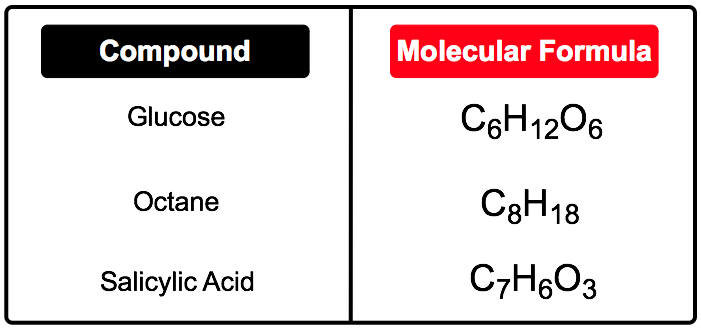Clutch Prep is now a part of Pearson
Ch.6 Chemical Reactions & QuantitiesWorksheetSee all chapters

# Molecular Formula

See all sections
Sections
Empirical Formula
Molecular Formula
Calculating Molar Mass
Mole Concept
Mass Percent
Stoichiometry
Limiting Reagent
Percent Yield

The molecular formula gives the actual number of atoms.

###### Molecular Formula

Concept #1: Molecular FormulaExample #1: After a workout session, lactic acid (M = 90.08 g/mol) forms in muscle tissue and is responsible for muscle soreness. Elemental analysis shows that this compound contains 40% C, 6.7% H and 53.3% O. Determine the molecular formula.

Practice: What is the molecular formula for the following compound?

Empirical Formula: NPCl                    Molar Mass: 347.64 g/mol

Practice: Cortisol (MW = 362.47 g/mol), a known steroid hormone, is found to contain 69.6% carbon, 8.34% hydrogen, and 22.1% oxygen by mass. What is its molecular formula?

Practice: Elemental analysis of a pure compound indicated that the compound had 72.2% C, 8.50% H and the remainder as O. If 0.250 moles of the compound weighs 41.55 g, what is the molecular formula of the compound?